SkyCivドキュメント

SkyCivソフトウェアのガイド - チュートリアル, ハウツーガイドと技術記事

1. ホーム
2. TechNotes
3. 読み込み中
4. 構造3Dで偏心点荷重を適用する方法

# 構造3Dで偏心点荷重を適用する方法

## An introduction to eccentric point loads in structural 3D modeling and analysis

When modeling a structure in SkyCiv Structural 3D, メンバーと接続は、メンバーのノードとラインで簡素化されます. ノード間のこれらの線は、単純さと連続性のために、常に各メンバーの重心を通過します. 実際には, メンバーに作用する荷重がその重心によって正当化できない場合に状況が発生します, これは偏心荷重です. Engineers need to think about eccentric loads when designing members because the addition of cross-sectional rotation, または ねじれ, できる, そしておそらくそうなるでしょう, 断面の限界状態に影響を与える.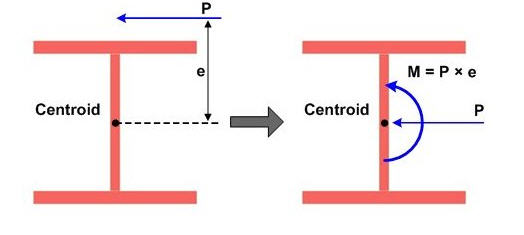ソース: http://manual.midasuser.com

Lets take a look at an example in SkyCiv Structural 3D and apply an eccentric point load two different ways. 最初, lets assume we have a W14x22 beam that is 15 feet long with an eccentric point load at midspan of 10 キップ, 演技 12 inches away from the centroid. We will assume that the load is acting downward (-Y direction) and to the left side (+Z direction) of the member. また, 自重がオフであると仮定します, 簡単にするために.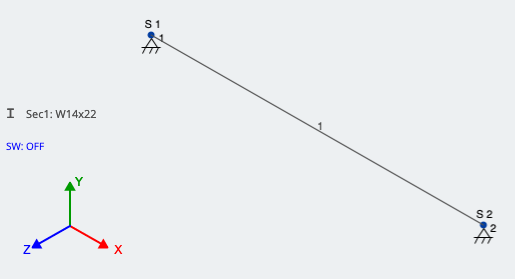$${P} = {10} キップ) \({e} = 12 inches = 1 foot$$

## 方法 1: Accounting for eccentricity by applying a Moment

$${M} = {P}*{e}$$

$${M} = 10 キップ * 1 foot = 10 kip-ft$$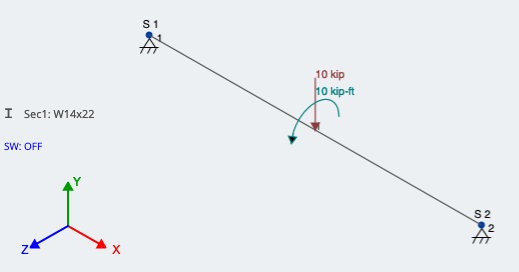## 方法 2: Using rigid links

Another method is to use rigid links. リジッドリンク are thought of as imaginary members that rotate and translate with whatever its connected to. They do not deflect between their nodes and are entirely stiff. Rigid Links are identified in the 3D modeling space as light grey and have a “R” next to them, 図に示すように 4. Because they are used more for connecting elements and loads, they do need a size or section ID.

2 つのノード間のメンバーを作成/描画する, それをリジッド リンクとして割り当てます. You can do this by pressing the 高度な switch in the member window, その後に行きます タイプ and changing it to リジッドリンク. 適用後, the member should look as described above. With one end signifying the actual location of the eccentric load and the other end connected to the member in the perpendicular direction, the load can finally be applied. This is shown in Figure 4; the red arrow is pointing to the Rigid Link: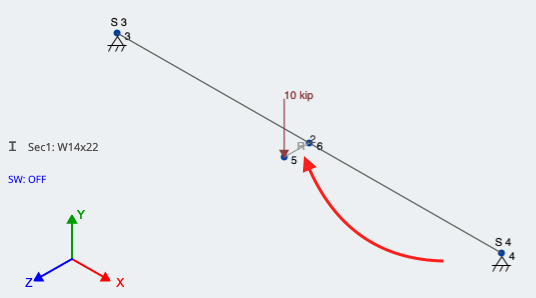## Final Comparison and Analysis:

Lets run a Linear Static Analysis and look at the results. We should be seeing the downward force of 10 kips in addition to a torsional component at the loading location. 両方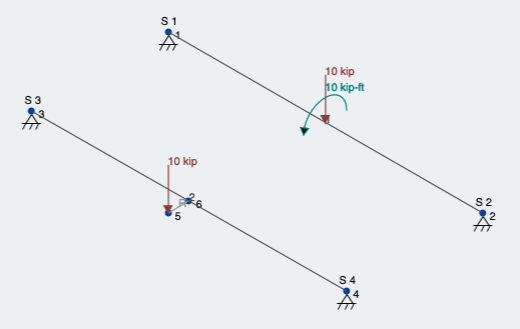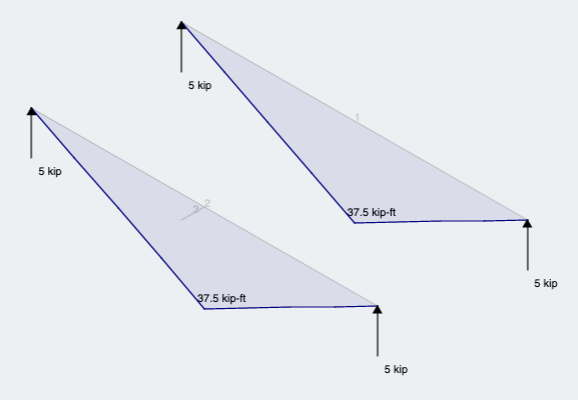As expected we see what we would expect from the same magnitude load and location along the member, but through the centroid.

その後, due to the eccentricity, we can observe that both members give the same result and show that the member is ALSO experiencing torsion (図 7):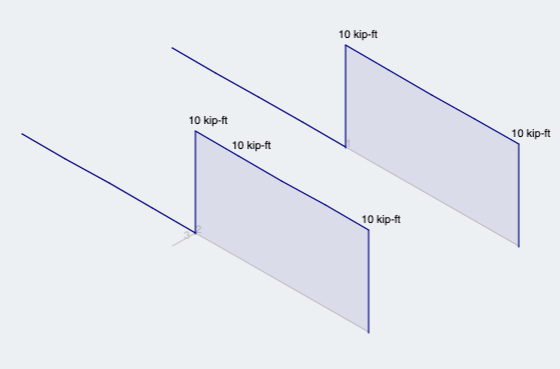トレバーソリー

ベン (民事)